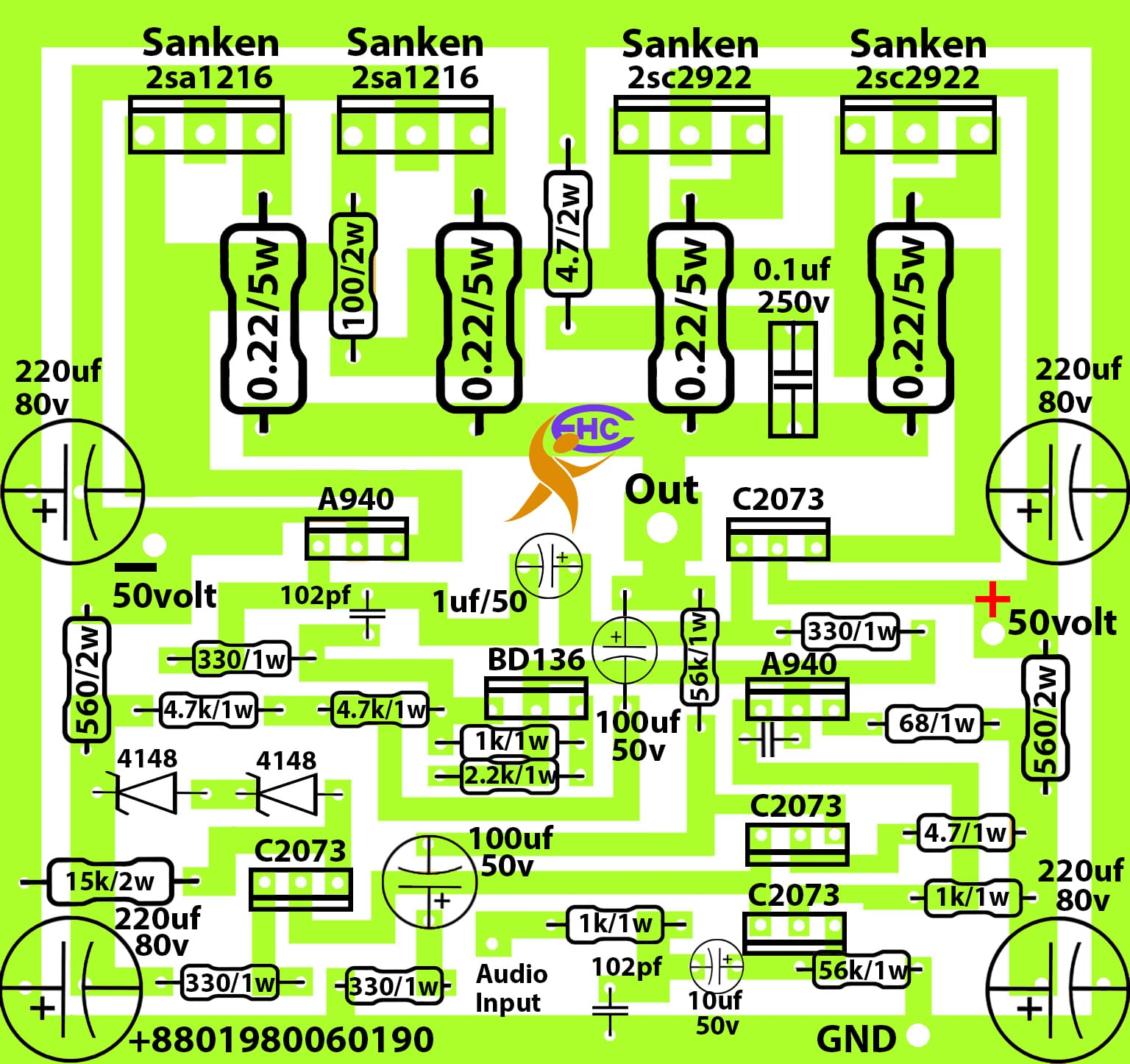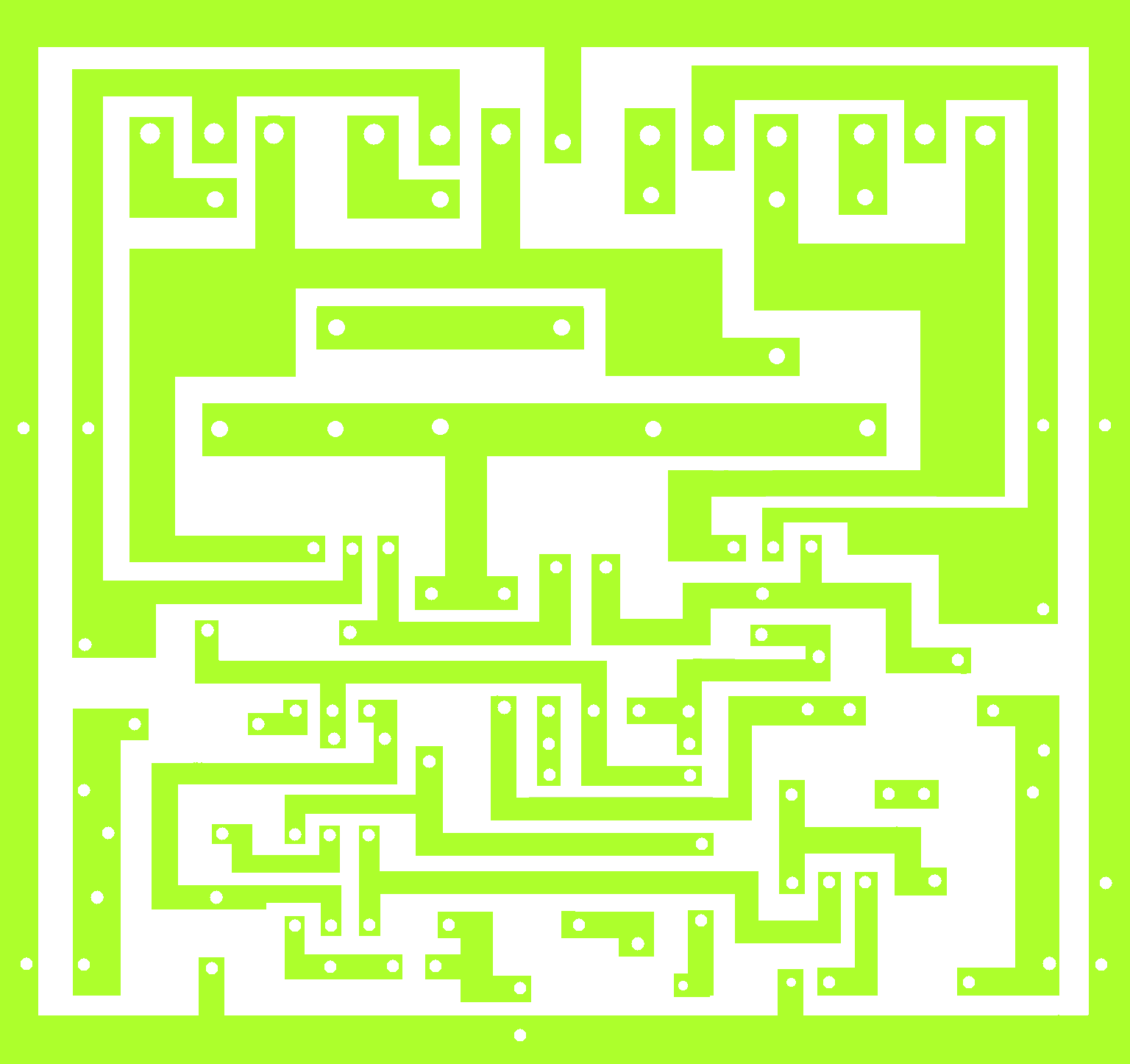# how to make sanken amplifier?

## how to make sanken amplifier:

This is an audio amplifier circuit diagram. this circuit has used sanken transistor. 2sc2922 and 2sa1216 transistors are used in this circuit. 4 transistors were used. 2 transistors are 2sc2922 and another 2sa1216. 2sc2922 is an NPN transistor. and 2sa1216 is a PNP transistor. This transistor can take 180 voltage maximum. collector amperes is 17 amperes. base amperes is 5 amperes. this transistor can make 200 watts.we can use 5 x 4 = 20 amperes maximum. and voltage is 50-0-50. We know that Voltage X ampere = Watts. so, 50×20=1000 watts. maximum 1000 watts can make this circuit. but we can’t use 20 amperes because driver transistor is A940 and C2073 transistor can’t take 20 amperes. next post I will make a circuit diagram to making mega watts. this circuit needs dual voltage. negative positive and ground voltage needs in this circuit.On our website, you can get many important circuit diagrams. not only amplifier but also invertervoltage controller. transistor amplifier. STK amplifier. Class-D amplifier. bass circuit/ low pass filter